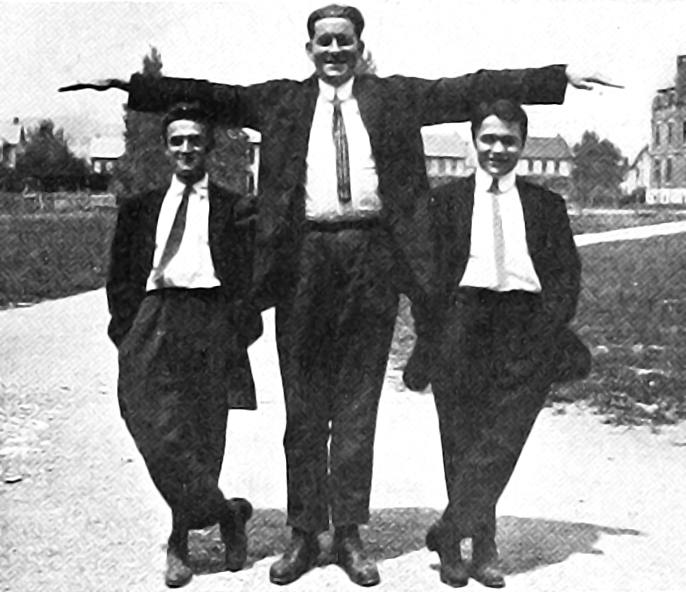# ELBO

## Evidence lower bound, variational free energy etc

$$\renewcommand{\Ex}{\mathbb{E}} \renewcommand{\dd}{\mathrm{d}} \renewcommand{\kl}{\operatorname{KL}} \renewcommand{\H}{\mathbb{H}} \renewcommand{\vv}{\boldsymbol{#1}} \renewcommand{\pd}{\partial}$$On using the most convenient probability metric (i.e. KL divergence) to do variational inference.

There is nothing novel here. But everyone who is doing variational inference has to work through this just once, and I’m doing so here.

Yuge Shi’s introduction is the best short intro that gets to state-of-the-art. The canonical intro is Matthews (2017) who did a thesis on it. Murphy (2012) sec 21.2 is also pretty good.

We often want a variational approximation to the marginal (log-)likelihood $$\log p_{\theta}(\vv{x})$$ (a.k.a. “evidence”) for some probabilistic model with observations $$\vv{x},$$ unobserved latent factors $$\vv{x}$$, model parameters $$\mathbb{\theta}$$ and variational parameters $$\phi$$.

Here’s one. The steps are all elementary, although realizing you would want to take those steps is not, IMO.

For convenience, we assume everything has a density with respect to some unspecifed dominating measure over $$\vv{x}$$ and $$\vv{z}$$, which is usually an OK assumption.1

\begin{aligned} \log p_{\theta}(\vv{x}) &=\log (p_{\theta}(\vv{x})) \Ex_{ q_{\phi}(\vv{z}\sim\vv{z}|\vv{x})}\left[ 1 \right] \\ &=\Ex_{ q_{\phi}(\vv{z}\sim\vv{z}|\vv{x})}\left[ \log (p_{\theta}(\vv{x})) \right]\\ &=\Ex_{ q_{\phi}(\vv{z}\sim\vv{z}|\vv{x})}\left[ \log \left( \frac{ p_{\theta}(\vv{x},\vv{z}) }{ p_{\theta}(\vv{z}|\vv{x}) } \right) \right]\\ &=\Ex_{ q_{\phi}(\vv{z}\sim\vv{z}|\vv{x})}\left[ \log\left( \frac{ q_{\phi}(\vv{z}|\vv{x})p_{\theta}(\vv{x},\vv{z}) }{ p_{\theta}(\vv{z}|\vv{x})q_{\phi}(\vv{x}|\vv{x}) }\right) \right]\\ &=\Ex_{ q_{\phi}(\vv{z}\sim\vv{z}|\vv{x})}\left[ \log \left(\frac{ q_{\phi}(\vv{z}|\vv{x}) }{ p_{\theta}(\vv{z}|\vv{x}) } + \frac{ p_{\theta}(\vv{x},\vv{z}) }{ q_{\phi}(\vv{z}|\vv{x}) }\right) \right]\\ &=\Ex_{ q_{\phi}(\vv{z}\sim\vv{z}|\vv{x})}\left[\log\left(\frac{ q_{\phi}(\vv{z}|\vv{x}) }{ p_{\theta}(\vv{z}|\vv{x}) }\right) \right] \\ &\qquad+\Ex_{ q_{\phi}(\vv{z}\sim\vv{z}|\vv{x})}\left[ \log\left(\frac{ p_{\theta}(\vv{x},\vv{z}) }{ q_{\phi}(\vv{z}|\vv{x}) }\right) \right] \nonumber\\ &=\kl_{\vv{z}}( q_{\phi}(\vv{z}|\vv{x}) \| p_{\theta}(\vv{z}|\vv{x}) ) +\mathcal{L}(\vv{x},\theta,\phi) \end{aligned}

$$\mathcal{L}(\vv{x},\theta,\phi):=\Ex_{ q_{\phi}(\vv{z}\sim\vv{z}|\vv{x})}\left[\log\left(\frac{ p_{\theta}(\vv{x},\vv{z}) }{ q_{\phi}(\vv{z}|\vv{x})}\right) \right]$$ is called the free energy or Evidence Lower Bound.

We can understand this as a decomposition of the total marginal evidence into two parts which are sort-of-interpretable. $$\kl_{\vv{z}}( q_{\phi}(\vv{z}|\vv{x}) \| p_{\theta}(\vv{z}|\vv{x}))$$ represents the cost of approximating the exact $$\theta$$ distribution over latents with the $$\phi$$ distribution. We can’t actually evaluate this in general but for fancy enough $$q_{\phi}$$ it could be small.

The other bit, $$\mathcal{L}(\vv{x},\theta,\phi)$$, represents an objective we can actually maximise, which motivates a whole bunch of technology. We can stop there and think of it as just that, or we can break it up further. The traditional next step if we want to decompose further is to observe that

\begin{aligned} \mathcal{L}(\vv{x},\theta,\phi) &=\Ex_{ q_{\phi}(\vv{z}\sim\vv{z}|\vv{x})}\left[\log\left(\frac{ p_{\theta}(\vv{x},\vv{z}) }{ q_{\phi}(\vv{z}|\vv{x}) } \right) \right]\\ &=\Ex_{ q_{\phi}(\vv{z}\sim\vv{z}|\vv{x})}\left[\log\left(\frac{ p_{\theta}(\vv{x}|\vv{z})p_{\theta}(\vv{z}) }{ q_{\phi}(\vv{z}|\vv{x}) } \right) \right]\\ &=\Ex_{ q_{\phi}(\vv{z}\sim\vv{z}|\vv{x})}\left[\log(p_{\theta}(\vv{x}|\vv{z})) \right] -\Ex_{ q_{\phi}(\vv{z}\sim\vv{z}|\vv{x})}\left[\log\left(\frac{ q_{\phi}(\vv{z}|\vv{x}) }{ p_{\theta}(\vv{z}) } \right) \right]\\ &=\underbrace{\Ex_{ q_{\phi}(\vv{z}\sim\vv{z}|\vv{x})}\left[\log(p_{\theta}(\vv{x}|\vv{z})) \right]}_{\text{Expected log likelihood}} - \underbrace{\kl_{\vv{z}}( q_{\phi}(\vv{z}|\vv{x}) \| p_{\theta}(\vv{z}))}_{\text{KL of approx posterior update}} \end{aligned}

This suggests we might intuit maximising the ELBO as maximising the data-conditional likelihood of the latents whilst penalising them for diverging too far from the prior on those latents. I find a marginal prior on the latents to be a weird concept here and this particular formulation makes my head hurt.

Yuge Shi, summarising Hoffman and Johnson (2016) and Mathieu et al. (2019) observes that we can break this down in a way which makes the per-observation latents into a coding problem. Suppose we index our $$N$$ observations by $$n$$, and they are independent. Then we can write this bad boy using the marginal $$q(\vv{z})$$.

\begin{aligned} \mathcal{L}(\vv{x},\theta,\phi) &= \underbrace{\left[ \frac{1}{N} \sum^N_{n=1} \Ex_{q(z_n\mid x_n)} [\log p(x_n \mid z_n)] \right]}_{\color{#4059AD}{\text{(1) Average reconstruction}}} - \underbrace{(\log N - \Ex_{q(z)}[\H[q(x_n\mid z)]])}_{\color{#EE6C4D}{\text{(2) Index-code mutual info}}} \notag \\ &\quad + \underbrace{\kl(q(z)\mid p(z))}_{\color{#86CD82}{\text{(3) KL between q and p}}} \notag \\ \end{aligned} $$\H\left[p(\vv{z}) \right] \triangleq-\Ex_{p(\vv{z})} \left[\log p(\vv{z})\right]$$ is entropy.

Another way to rewrite the ELBO is as $\mathcal{L}(\vv{x},\theta,\phi)=\Ex_{q_{\phi}(\vv{z} \mid \vv{x})}\left[\log p_{\theta}(\vv{z}, \vv{x})\right]+\H\left[q_{\phi}(\vv{z} \mid \vv{x})\right].$ The log joint $$\log p_{\theta}(z, x)$$ what physics people call the “negative energy”. This version highlights that a good posterior approximation $$q_{\phi}(z \mid x)$$ must assign most of its probability mass to regions of low energy (i.e. high joint probability). At the same time the entropy term in the ELBO prevents $$q_{\phi}(z \mid x)$$ from collapsing to an atom, unlike in, say, an MAP estimate.

Next thing, Importance weighted sampling in variational inference. Also a recommendation from Yuge Shi, see Adam Kosiorek’s What is wrong with VAEs? which finds autoencoders via importance sampling.

## Bethe free energy

Everything so far as been about Helmholtz Free Energy. In graphical models graphs we are concerned with a related, more general (?) free energy called Bethe free energy [J. S. Yedidia, Freeman, and Weiss (2005);Jonathan S. Yedidia, Freeman, and Weiss (2000); WainwrightGraphical2008].

## Incoming

1. Although in machine learning we tend to assume the dominating measure is Lebesgue, which as Matthews (2017) shows, can get you in to trouble.↩︎

### No comments yet. Why not leave one?

GitHub-flavored Markdown & a sane subset of HTML is supported.